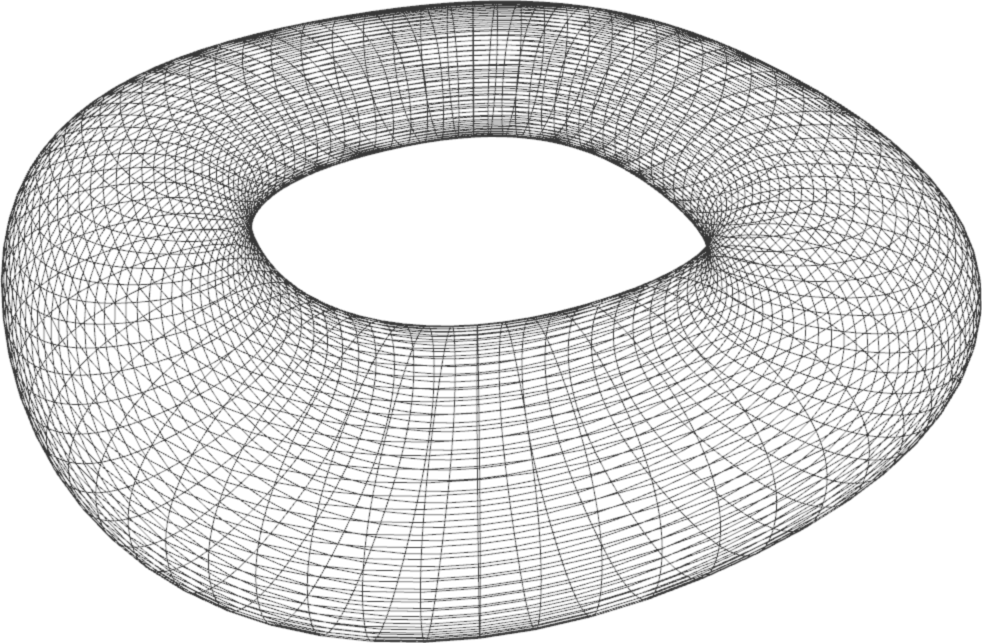TP9 submission deadline is Thursday, 13/04/2017 at 23:59. The TP on Friday 14/04 will start at 13:30 (instead of 15:15).

# TP7 : B-spline Surfaces

24 March 2017 in

## B-spline curves revisited

Back in TP3, we were working with B-spline curves. A B-spline curved is defined by the following:

## Tensor product B-spline surfaces

A B-spline surface is defined via

B-spline surface is defined for $(u,v) \in [u_{du},u_{m+1}[ \times [v_{dv},v_{n+1}[$ as

where $N$ are the basis functions (see TP3).

## Surface Patches

Recall that a B-spline curve is made from many smaller pieces, defined over parameter intervals $[t_i,t_{i+1}]$. Analogically, a B-spline surface consists of patches, each defined over a parameter rectangle $[u_i,u_{i+1}] \times [v_j,v_j+1]$ :

## Algorithm

We’ll evaluate and display each patch individually. The algorithm is summarized in the following pseudocode.

# loop over all patches
for i in (du,du+1,...,m) :
for j in (dv,dv+1,...,n) :
# check if the patch is not degenerate
if not valid_patch :
continue
# loop over the samples of the current patch
# defined over [u_i,u_i+1] x [v_j,v_j+1]
for u in uniform_sampling( u_i, u_i+1, number_of_samples ) :
for v in uniform_sampling( v_j, v_j+1, number_of_samples ) :
compute_S(i,j,u,v) # a surface point on the current patch


## ToDo

1. Implement evaluation of B-spline surfaces. Test with the provided datasets (simple.bspline and torus.bspline).
2. Modify the knot vectors for the simple dataset. Experiment with various configurations. How does the surface change?
3. [Bonus] NURBS surfaces can be used to represent the unit sphere, the same way we used NURBS curves to represent the unit circle in TP3. Modify your implementation of B-spline surfaces to compute NURBS surfaces. Test with the hemisphere (hemi.nurbs) and the modified torus (torus.nurbs).
Note: full sphere control points, weights, and knots can be found in Representing a Circle or a Sphere with NURBS by David Eberly.